Like this site? Pledge support via Patreon!# List of maths words

Mathematical concepts, illustrated with photographs.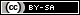## Ais forAcute

An acute angle is one that is smaller than 90 degrees. A 90 degree angle is a "normal" corner, like you find inside most houses and the corners of a piece of paper. Acute angles can also be described as sharp or pointy. An angle larger than 90 degrees is called obtuse.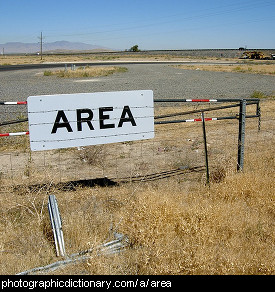## Ais forArea

An area is a part of any surface. Areas are usually separated from everything around them by some kind of boundary, such as a fence or a line drawn around them on a piece of paper. Area also describes the total size of that area. Different shapes have different formula for calculating how big they are.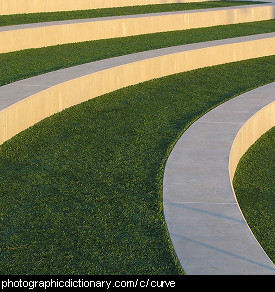## Cis forCurve

A curve is a line that bends to one side or the other along its length. The opposite of curved is straight.## Dis forDiameter

The diameter of a circle is the measurement across the circle at its widest point. The man in the picture has his arms across the diameter of the cut tree.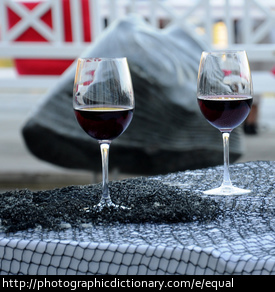## Eis forEqual

Two things are equal when there are no difference in the amounts of each thing. For example, two glasses each with equal amounts of wine in them.## His forHalf

This glass of water is half-full (some people would say half-empty). If you took two half-glasses of water and poured them together, you would get a full glass. A half is what you get when you cut something into two equal sized pieces.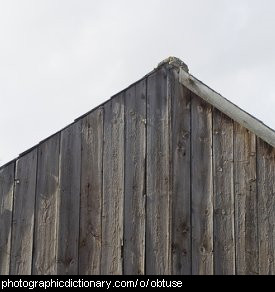## Ois forObtuse

An obtuse angle is one that is larger than 90 degrees. A 90 degree angle is a "normal" corner, like you find inside most houses and the corners of a piece of paper. An angle smaller than 90 degrees is called acute.## Ois forOdd

Something is odd if it is different to usual, or is a bit strange. In a group of things, something that is different is called the odd one out. The man in the picture is wearing odd socks. Mathematically, a number is odd if it is not a multiple of two.## Pis forParallel

Two lines are parallel if they are next to each other and are always the same distance apart. There are many parallel lines in buildings. The vertical lines the bricks make in the right side of the picture are parallel.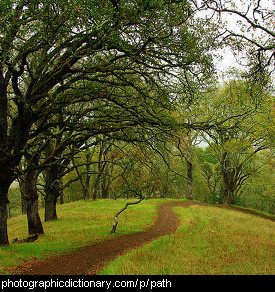## Pis forPath

A path is a small road that connects two places. Paths are usually for people or animals, not large vehicles. In math, a path is simply a way to connect two points, not necessarily in a straight line.